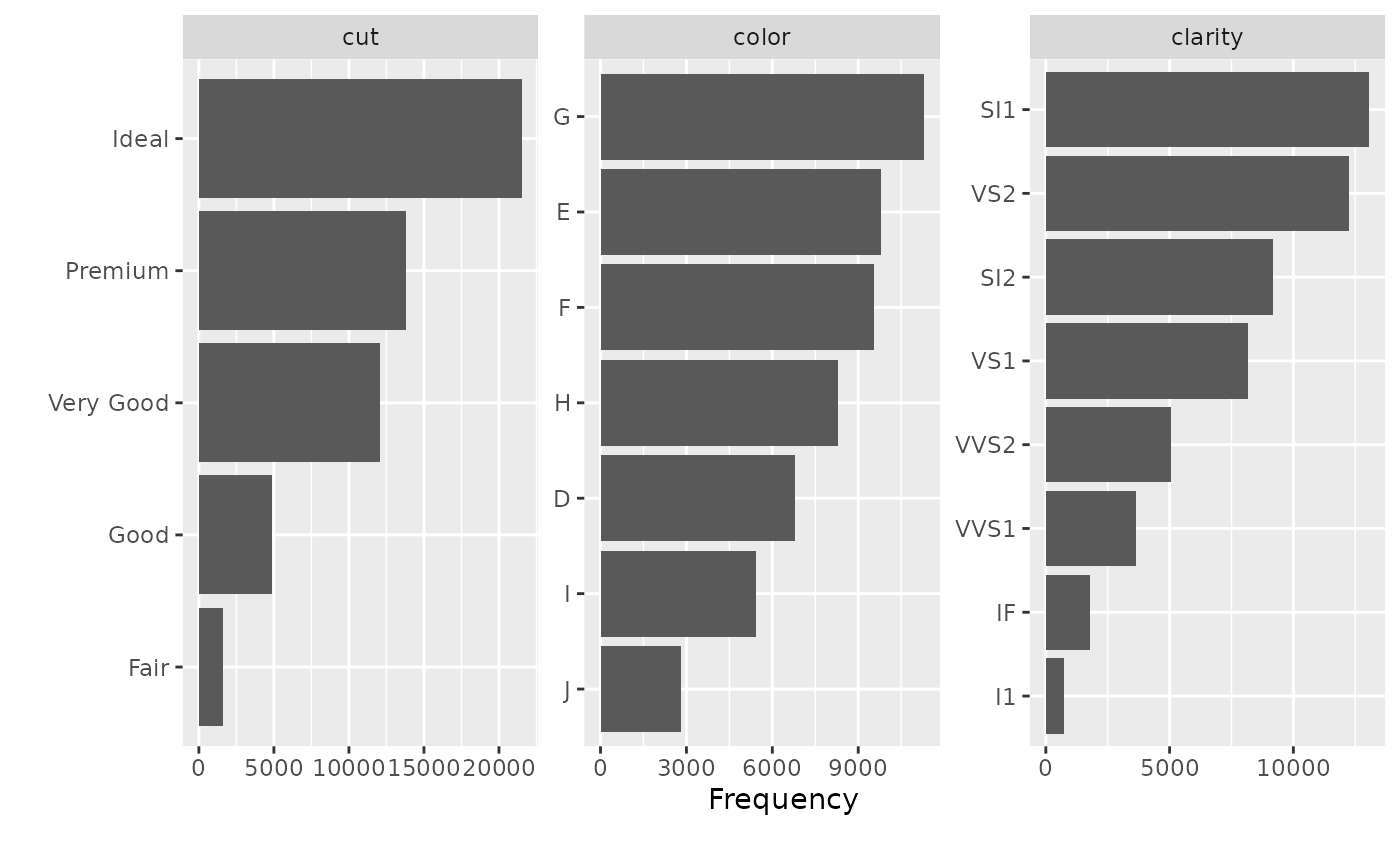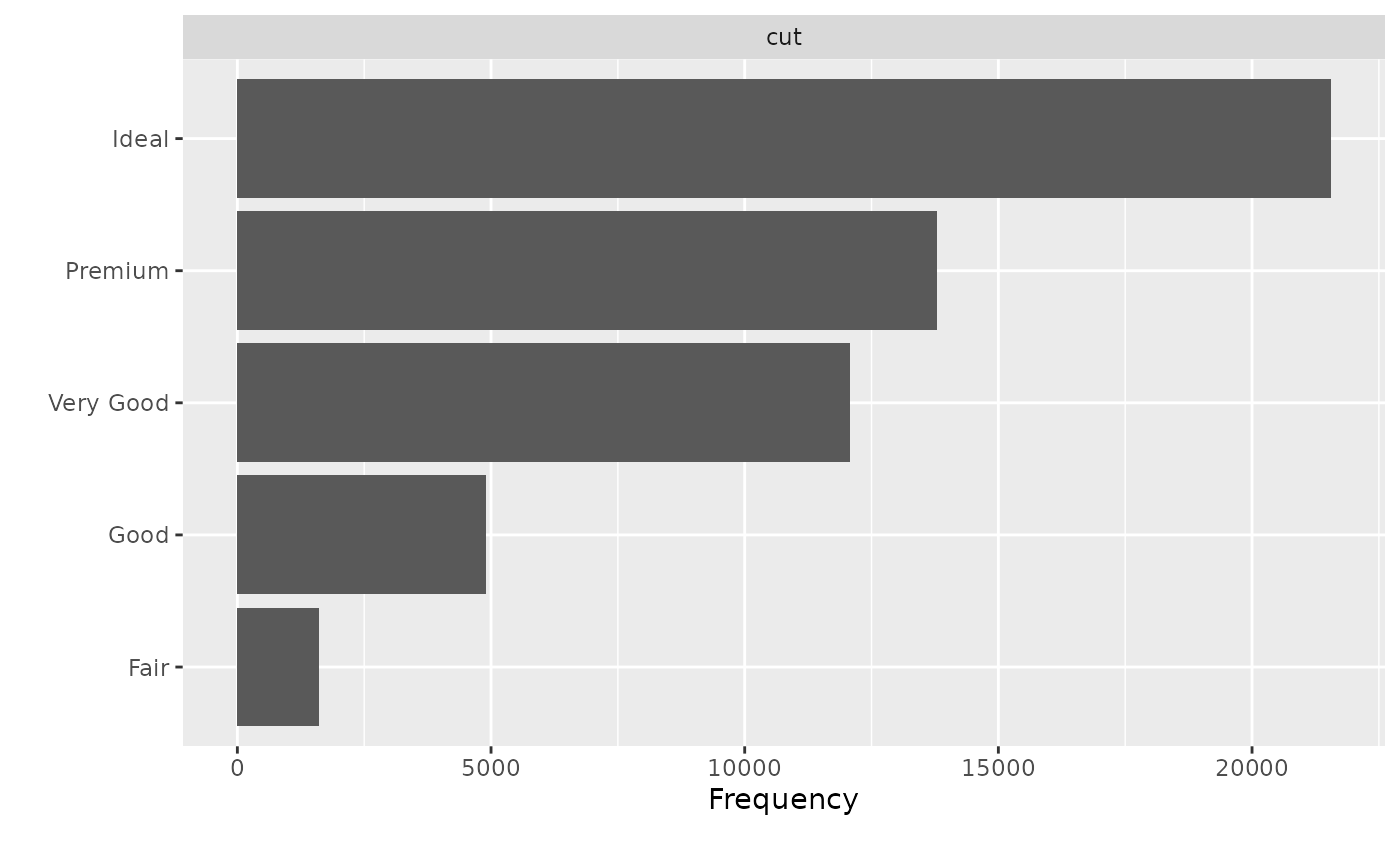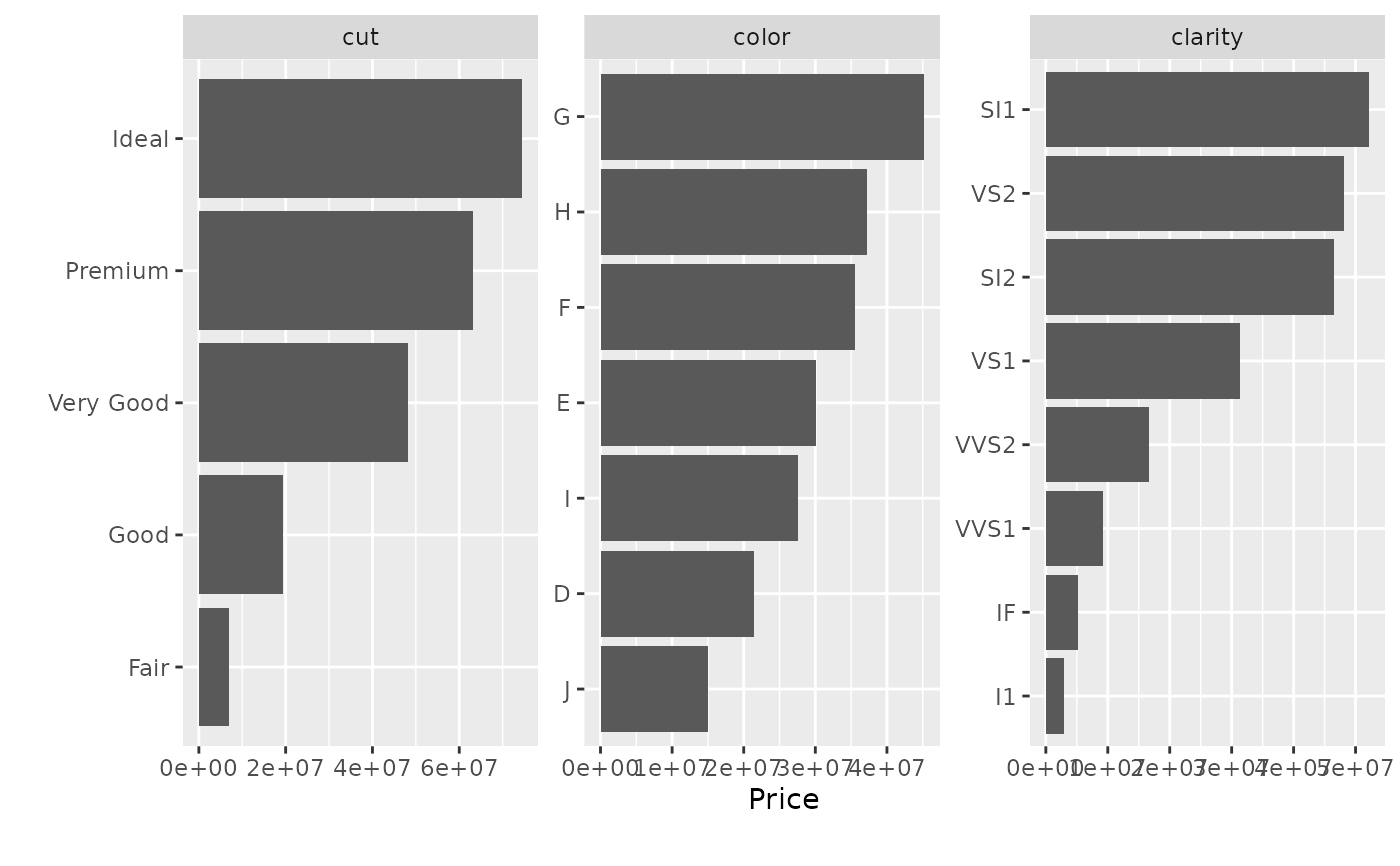Plot bar chart for each discrete feature, based on either frequency or another continuous feature.

plot_bar(data, with = NULL, maxcat = 50, order_bar = TRUE,
binary_as_factor = TRUE, title = NULL, ggtheme = theme_gray(),
theme_config = list(), nrow = 3L, ncol = 3L, parallel = FALSE)

## Arguments

data input data name of continuous feature to be summed. Default is NULL, i.e., frequency. maximum categories allowed for each feature. Default is 50. logical, indicating if bars should be ordered. Default is TRUE. treat binary as categorical? Default is TRUE. plot title complete ggplot2 themes. Default is theme_gray. a list of configurations to be passed to theme number of rows per page. Default is 3. number of columns per page. Default is 3. enable parallel? Default is FALSE.

## Value

invisibly return the named list of ggplot objects

## Details

If a discrete feature contains more categories than maxcat specifies, it will not be passed to the plotting function.

## Examples

# Plot bar charts for diamonds dataset
library(ggplot2)
plot_bar(diamonds)plot_bar(diamonds, maxcat = 5)#> 2 columns ignored with more than 5 categories.
#> color: 7 categories
#> clarity: 8 categories# Plot bar charts with price feature
plot_bar(diamonds, with = "price")